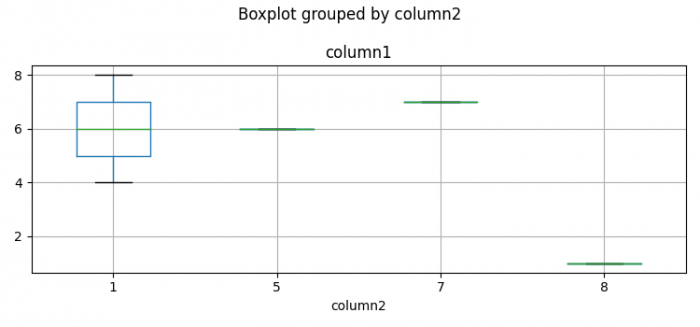# Boxplot stratified by column in Python Pandas

To create a boxplot stratified by column in Python class, we can take the following steps −

## Steps

• Set the figure size and adjust the padding between and around the subplots.

• Create a Pandas data frame of two-dimensional, size-mutable, potentially heterogeneous tabular data.

• Compute the histogram of a set of data.

• Create a boxplot startified by column.

• To display the figure, use show() method.

## Example

import pandas as pd
import numpy as np
from matplotlib import pyplot as plt

# Set the figure size
plt.rcParams["figure.figsize"] = [7.50, 3.50]
plt.rcParams["figure.autolayout"] = True

# Pandas dataframe
df = pd.DataFrame({"column1": [4, 6, 7, 1, 8], "column2": [1, 5, 7, 8, 1]})

# Compute the histogram
_, breaks = np.histogram(df.column1, bins=5)

# Create the boxplot
ax = df.boxplot(column='column1', by='column2')

# Display the plot
plt.show()

## Output

It will produce the following output −Updated on: 02-Feb-2022

274 Views